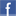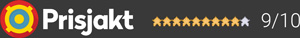#### Inga produkter tillagda!

Lägg en produkt i varukorgen så ser det lite roligare ut här ;)

#### Varukorg

0 kr# FocalSW1000 Be

39 990 krVälj utförande
39 990 kr
Beställningsvara (5-7 dagar för varor som finns i lager hos leverantören)
Fri frakt!

The SW 1000 Be subwoofer is the redesigned version of the Electra Be subwoofer to ensure compatibility with the other loudspeakers in the Sopra line.
It is equipped with a 13" (33cm) "W" cone speaker driver (high rigidity for no distortion, even at high volumes), a high-power 600 W RMS BASH® amplifier and a front laminar port (no dynamic bass compression).
The 24-bit digital processor (DSP) offers a large control panel with settings for adapting the sub to various listening rooms. The SW 1000 Be offers very high performance with a bass cut-off frequency of 18 Hz and an SPL of 118 dB.

Several SW 1000 Be subwoofers can be linked up.

Aktiv Subwoofer

1 x 131/2” W-Bas

600W Förstärkare

24bitars DSP

Garanti i 24 månaderDela på Facebook

#### Frågor? Ring 031-241600eller maila //<![CDATA[ var l=new Array(); l = '>'; l = 'a'; l = '/'; l = '<'; l = '|109'; l = '|111'; l = '|99'; l = '|46'; l = '|110'; l = '|114'; l = '|97'; l = '|108'; l = '|108'; l = '|97'; l = '|107'; l = '|100'; l = '|117'; l = '|106'; l = '|108'; l = '|64'; l = '|111'; l = '|102'; l = '|110'; l = '|105'; l = '>'; l = '"'; l = '|109'; l = '|111'; l = '|99'; l = '|46'; l = '|110'; l = '|114'; l = '|97'; l = '|108'; l = '|108'; l = '|97'; l = '|107'; l = '|100'; l = '|117'; l = '|106'; l = '|108'; l = '|64'; l = '|111'; l = '|102'; l = '|110'; l = '|105'; l = ':'; l = 'o'; l = 't'; l = 'l'; l = 'i'; l = 'a'; l = 'm'; l = '"'; l = '='; l = 'f'; l = 'e'; l = 'r'; l = 'h'; l = ' '; l = 'a'; l = '<'; for (var i = l.length-1; i >= 0; i=i-1) { if (l[i].substring(0, 1) === '|') document.write("&#"+unescape(l[i].substring(1))+";"); else document.write(unescape(l[i])); } //]]>